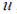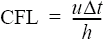# TIME_ INCREMENT

Specifies the time increment values and control parameters.

AcuSolve Command

## Syntax

TIME_INCREMENT {parameters}

## Qualifier

This command has no qualifier.

## Parameters

initial_time_increment or init_dt (real) >=0 [=0]
Initial value of the time increment for auto time increment control, or the constant value of the time increment for a fixed time increment. If zero, it is set to the largest time increment that satisfies the initial_cfl_number condition.
previous_time_increment (real) >=0 [=0]
Time increment of the previous time step. If zero, it is automatically set for restart runs.
flow_time_increment (real) >=0 [=0]
Time increment associated with the flow field. Needed for frozen flow simulations.
auto_time_increment or auto_dt (boolean) [=on]
Flag specifying whether or not to automatically control the time increment.
local_time_increment or local_dt (boolean) [=off]
Flag specifying whether or not to use the local time stepping algorithm. If on, a different time increment is used at each quadrature point. This option should only be used for steady-state solutions.
min_time_increment or min_dt (real) >=0 [=0]
Minimum time increment when using the auto time increment option.
max_time_increment or max_dt (real) >=0 [=0]
Maximum time increment when using the auto time increment option.
cfl_control (boolean) [=on]
Flag specifying whether or not to control the time increment based on the CFL number. This option is used only with the auto time increment option.
cfl_number or cfl (real) >0 [=100]
CFL number used with the CFL control and local time increment options.
min_cfl_number or min_cfl (real) >=0 [=0]
Minimum value of the CFL number when using the auto time increment option with CFL control.
initial_cfl_number or init_cfl (real) >=0 [=1]
Initial value of the CFL number. This option is used to determine an initial time increment when initial_time_increment is zero.
time_increment_decrease_factor or dt_dec_fct (real) >0 <=1 [=0.25]
Factor used to reduce the time increment when a time step needs to be re-done due to lack of convergence. This option is used only with the auto time increment option.
time_increment_increase_factor or dt_inc_fct (real) >= 1 [=1.25]
Factor used to increase the time increment when the previous time step is converged. This option is used only with the auto time increment option.
time_increment_increase_delay or dt_inc_delay (integer) >=0 [=4]
Number of time steps to wait, after a time increment reduction and re-do of a time step, before allowing the time increment to increase. This option is used only with the auto time increment option.
min_time_increment_ratio or min_dt_ratio (real) >=0 <=1 [=0.1])
The minimum ratio of two consecutive time increments allowed when the previous time step is repeated with a smaller time increment. This option is used only with the auto time increment option.
multiplier_function (string) [=none]
User-given name of the multiplier function for scaling the time increment or CFL number, as appropriate. If none, no scaling is performed.

## Description

This command specifies the time increment options for the time marching solution of a problem. Two time increment strategies are available: fixed and auto time increment. In addition, a local time stepping method may be used with either strategy. A fixed time increment strategy with a global time stepping method may be set up as follows:
TIME_INCREMENT {
initial_time_increment      = 1.0
auto_time_increment         = off
local_time_increment        = off
}
Here a fixed time increment of initial_time_increment is used for all time steps. If the time increment is set to zero, such as in
TIME_INCREMENT {
initial_time_increment      = 0
auto_time_increment         = off
local_time_increment        = off
initial_cfl_number          = 10
}

then the initial conditions are made to satisfy the boundary conditions of the first time step and the maximum time increment that satisfies the initial_cfl_number is computed. This time increment is used for all time steps. If this time increment happens to be zero, then a time increment of one is used. Note that the CFL number is defined based on the velocity,, time increment, $\text{Δ}$ t and a measure of element size, h, as follows:When local time stepping with fixed time increment strategy is used, such as in:
TIME_INCREMENT {
initial_time_increment      = 1
auto_time_increment         = off
local_time_increment        = on
cfl_number                  = 100.
}

a different time increment is used at each quadrature point of each element. This time increment is such that the CFL number at the quadrature is equal to cfl_number. In this case, the initial_time_increment has little meaning. Note that the local time stepping method may only be used for steady-state solutions.

An auto time increment strategy with a global time stepping method may be set up as follows:
TIME_INCREMENT {
initial_time_increment              = 1.0
auto_time_increment                 = on
local_time_increment                = off
min_time_increment                  = 0.001
max_time_increment                  = 10.0
cfl_control                         = on
cfl_number                          = 100.
min_cfl_number                      = 1.
time_increment_decrease_factor      = 0.25
time_increment_increase_factor      = 1.25
time_increment_increase_delay       = 4
min_time_increment_ratio            = 0.1
}

Here an initial time increment of initial_time_increment is used. The time increment is allowed to increase up to max_time_increment and decrease to min_time_increment. Every time step may increase the time increment by no more than a factor of time_increment_increase_factor. This growth restriction is also imposed on the CFL number of the time steps. If the cfl_control option is used, the time increment value is restricted so that the CFL number may not exceed cfl_number nor fall below min_cfl_number at all quadrature points in the problem.

A time step may be redone with a new time increment reduced by a factor of time_increment_decrease_factor if any of the following conditions occur:
• A transient problem is being solved (see parameter mode of ANALYSIS command) and the last set of convergence measures of the stagger loop are greater than the stagger_convergence_tolerance parameter of the TIME_SEQUENCE command. This condition is ignored if the time increment is already at min_time_increment.
• The computed time increment to be used for the next time step is less than min_time_increment_ratio times the one used for the current time step. This condition typically occurs when the velocity field increases very rapidly, indicating some kind of numerical instability.
• Any of the algorithms in the code detects errors that may cause severe inaccuracies in the problem. This condition is ignored if the time increment is already at min_time_increment.

Once a time step is repeated with a reduced time increment, a minimum of time_increment_increase_delay time steps are solved before the time increment is allowed to increase again.

An auto time increment strategy with local time stepping method may be set up similarly to the above case, with local_time_increment turned on:
TIME_INCREMENT {
initial_time_increment              = 1.0
auto_time_increment                 = on
local_time_increment                = on
min_time_increment                  = 0.001
max_time_increment                  = 10.0
cfl_control                         = on
cfl_number                          = 100.
min_cfl_number                      = 1.
time_increment_decrease_factor      = 0.25
time_increment_increase_factor      = 1.25
time_increment_increase_delay       = 4
min_time_increment_ratio            = 0.1
}

In contrast to the fixed time increment case, a different time increment is used at each quadrature point of each element. This time increment is such that the CFL number at the quadrature is equal to a running CFL number. This CFL number is controlled along with the time increment, starting with the initial_cfl_number. Again, this option should only be used for steady-state problems.

The flow_time_increment parameter is needed when a previously computed flow field is used for the simulation of scalar fields. Typically the flow field is steady and the scalar simulation is transient, but any combination of steady/transient simulations is allowed. The flow field must be computed with a global time stepping strategy and the value supplied for flow_time_increment must be equal to the value of the time increment when the flow field was saved. If a different value for flow_time_increment is used then the conservation properties of the scalar field will be lost. For example, a steady flow field may be solved with
ANALYSIS {
}
EQUATION {
flow                                = navier_stokes
turbulence                          = spalart_allmaras
}
TIME_INCREMENT {
initial_time_increment              = 10.0
auto_time_increment                 = off
local_time_increment                = off
}
...
If AcuTrans is used to create nodal solution files, then a transient thermal simulation can then be performed using
ANALYSIS {
title                               = "Transient thermal solution"
type                                = transient
}
EQUATION {
}
NODAL_INITIAL_CONDITION( velocity ) {
}
NODAL_INITIAL_CONDITION( pressure ) {
}
NODAL_INITIAL_CONDITION( eddy_viscosity ) {
...